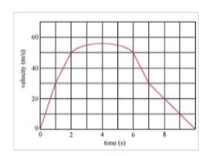# Problem: The graph in the figure shows the velocity v of a sports car as a function of time t. Find the maximum acceleration amax of the car.

###### FREE Expert Solution

Acceleration:

$\overline{){\mathbf{a}}{\mathbf{=}}\frac{\mathbf{∆}\mathbf{v}}{\mathbf{∆}\mathbf{t}}}$

The slope of the graph is the acceleration.

81% (151 ratings)###### Problem Details

The graph in the figure shows the velocity v of a sports car as a function of time t.Find the maximum acceleration amax of the car.